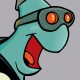Hello,

I am using a turtlebot 2 with ros-groovy. I have successfully wrote a publisher that takes the base_link and broadcasts that info. I am working on writing a broadcaster for the base_footprint. Also my base_link broadcaster works perfect. That is why I just followed the same format for that. But I don't understand why this logic is happening.

The base_foot print broadcaster seems to be messing up though. The broadcaster is able to keep the correct distance but when I start turning the robot things go bad. What goes wrong is that as the robot starts turning the base_footprint frame starts revolving around the fixed map frame (0,0,0). What I would expect is that whenever the turtlebot turns in the z direction that the base_footprint stays with the robot model on rviz like it is suppose to. If anyone could help it would be appreciated.I have 2 examples there of what Is going on so you can get an idea.

while(ros::ok()){
tf::StampedTransform transformFP; //for the base_footprint
current_time = ros::Time::now();

//This is for the base_link frame which works great this block of code just gets the transform and that transform is

try {
}
catch (tf::TransformException &ex) {
ROS_ERROR("%s",ex.what());
}
yaw =   tf::getYaw(transform.getRotation());
v.setValue(transform.getOrigin().x() , transform.getOrigin().y() , transform.getOrigin().z());

q.setRPY(0.0, 0.0, yaw);

double th = yaw;
geometry_msgs::Quaternion odom_quat = tf::createQuaternionMsgFromYaw(th);
transformConverter.transform.translation.x = transform.getOrigin().x();
transformConverter.transform.translation.y = transform.getOrigin().y();
transformConverter.transform.translation.z = transform.getOrigin().z();
transformConverter.transform.rotation = odom_quat;

/*This is where the base_footprint is being created I also believe this is where the code is messing up.
As the robot turns in the yaw angle the position of the base_footprint starts translating into a + or - direction but the  distance is kept . As you can see in the picture that is what happens when the robot turns in a 180 degrees. The base_footprint gets shifted in 180 degrees  This is following the same exact code as the base_link that works fine as well*/
try {
listener.waitForTransform("base_footprint", "odom", ros::Time(0), ros::Duration(10.0));
listener.lookupTransform("base_footprint", "odom", ros::Time(0), transformFP);
}
catch (tf::TransformException &ex) {
ROS_ERROR("%s",ex.what());
}

*/  double tryYaw = atan2( (2*transformFP.getRotation().w()*transformFP.getRotation().z() ),                           (transformFP.getRotation().w()*transformFP.getRotation().w() - transformFP.getRotation().z()*transformFP.getRotation().z()));       This is another equation I tried but it basically does the same exact stuff. */

//this is where the yaw angle gets calculated.
yaw2 =  tf::getYaw(transformFP.getRotation());
v.setValue(transformFP.getOrigin().x() , transformFP.getOrigin().y() , transformFP.getOrigin().z());

q ...
edit retag close merge delete

Please descrive what "go bad" means. What you expect to see and what you are actually seeing. Also documentation in your code as to what you want to happen would be helpful.

I have edited my post hopefully it's a little more clear. Thanks Mr. tfoote creator of the tf universe.

Also I put a description on the pictures but the are not coming up. The top picture is showing the turtlebot and the broadcasted base_footprint. The bottom picture is showing what happens when the turtlebot is going in positive direction on the y axis the distance of the broadcasted frame is kept

and so is the orientation. The top picture is what goes wrong when the robot turns.

Sort by » oldest newest most votedYour problem is that you're inverting the order of the publishing without inverting the matrix. You lookup the transform from base_footprint to odom, but you publish odom to leader_base_footprint. Inverting the direction requires inverting the transform.

Note you have the same problem with your base_link to base_footprint, but it's likely the identity or very small with out rotations, so you don't notice.

more

Thank you that actually fixed the problem. Now time for the Odom frame.